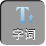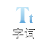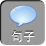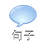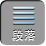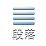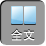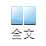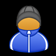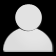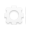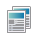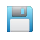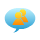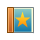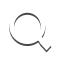-AA+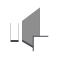1、已知：如图 1，矩形的对角线 ACBD相交于点 O，∠ AOB=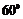AB= 5 cm，求 AC的长.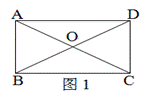AC= BD, OA=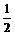AC, OB=BD,即 OA= OB.

∴△ AOB是等边三角形.

AO= AB= 5 (cm),

AC= 2 AO= 2× 5 cm= 10 cm.

2、已知：如图 2，在矩形 ABCD中，对角线 ACBD相交于 OAEBD，垂足为 E，∠ DAE：∠ BAE= 31，求∠ EAC的度数.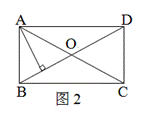∵∠ DAE：∠ BAE= 31

3BAE：∠ BAE=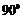，即 4BAE=,

∴∠ BAE=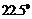.

∴∠ ABE=-BAE=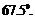AC= BD, OA= OC, OB= OD.

OA= OB,

∵∠ OAB=∠ ABE=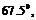∴∠ EAC=∠ OAB -BAE=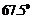-=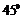.

3、如图 3,宽为 50 cm的矩形图案由 10个全等的小长方形拼成,其中一个小长方形的面积为________.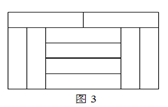4、已知：如图 4，在矩形 ABCD中，∠ B的平分线交 CDEEFAEBC于点 F.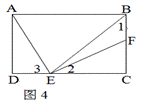∴∠ 1=∠ BEC,∴ EC= BC= AD.

∴∠ 2=∠ DAE.

∴△ ADE≌△ ECF (AAS). AE= EF.

5、如图 5，在△ ABC中，∠ C=, AC= BC,自 AB上任一点 P,作 PEBCE, PFACF, MAB的中点.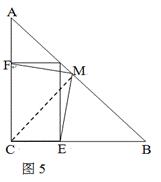CM= MB= MA,∴∠ B=∠ MCB,∠ A=∠ MCA.

PFAC, P EBC,∴∠ PFC==∠ PEC=∠ ACB.

∴四边形 PECF为矩形.

PE= FCPEAC,∴∠ BPE=∠ A==∠ B.

PE= EB= FC.

∴△ CMF≌△ BME, ME= MF.

∴△ MEF是等腰三角形.